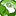# QlikView App Dev

Discussion Board for collaboration related to QlikView App Development.

Announcements
Action-Packed Learning Awaits! QlikWorld 2023. April 17 - 20 in Las Vegas: REGISTER NOW
cancel
Showing results for
Did you mean:Not applicable

## Color Code based on Inline Table utilizing two dimensions in that table

I currently utilize color coding of cells in a straight table by using background expressions for each expression. There are two different sets of ranges per expression; I control which set by way of the variable vSLAindicator (for toggling).  I'm thinking this can be simplified by creating an Inline Table with two sets of ranges, but haven't quite figured out how bust to structure this Table and what functions I could used to look up the range vales given they would be based on two dimensions in that table, i.e. some sort of set indicator and another to designate which expression these ranges apply to.

Background Expression associated to Expression 1

if(vSLAindicator = 0,
if(Sum(IF(RuntimeMins < 5,1,0))/count(Attempt)*100 > 29,RGB(3,197,255),
if(Sum(IF(RuntimeMins < 5,1,0))/count(Attempt)*100 >= 25 and Sum(IF(RuntimeMins < 5,1,0))/count(Attempt)*100 <= 29,RGB(154,232,108),
if(Sum(IF(RuntimeMins < 5,1,0))/count(Attempt)*100 >= 20 and Sum(IF(RuntimeMins < 5,1,0))/count(Attempt)*100 <= 25,Yellow(),
if(Sum(IF(RuntimeMins < 5,1,0))/count(Attempt)*100 < 20,LightRed(),
)))),
if(Sum(IF(RuntimeMins < 5,1,0))/count(Attempt)*100 >= 70,RGB(154,232,108),
if(Sum(IF(RuntimeMins < 5,1,0))/count(Attempt)*100 >= 50 and Sum(IF(RuntimeMins < 5,1,0))/count(Attempt)*100 <= 70,Yellow(),
if(Sum(IF(RuntimeMins < 5,1,0))/count(Attempt)*100 < 50,LightRed(),
))))

Background Expression associated to Expression 2

if(vSLAindicator = 0,
if(Sum(IF(RuntimeMins < 15,1,0))/count(Attempt)*100 > 73,RGB(3,197,255),
if(Sum(IF(RuntimeMins < 15,1,0))/count(Attempt)*100 >= 50 and Sum(IF(RuntimeMins < 15,1,0))/count(Attempt)*100 <= 73,RGB(154,232,108),
if(Sum(IF(RuntimeMins < 15,1,0))/count(Attempt)*100 >= 40 and Sum(IF(RuntimeMins < 15,1,0))/count(Attempt)*100 <= 50,Yellow(),
if(Sum(IF(RuntimeMins < 15,1,0))/count(Attempt)*100 < 40,LightRed(),
)))),
if(Sum(IF(RuntimeMins < 15,1,0))/count(Attempt)*100 >= 85,RGB(154,232,108),
if(Sum(IF(RuntimeMins < 15,1,0))/count(Attempt)*100 >= 70 and Sum(IF(RuntimeMins < 15,1,0))/count(Attempt)*100 <= 85,Yellow(),
if(Sum(IF(RuntimeMins < 15,1,0))/count(Attempt)*100 < 70,LightRed(),
))))

There are 2 more like these.

8 RepliesPartner - Creator III

Help me out, I'm not exactly sure what you are trying to achieve.

Can you give a simple example?Partner - Champion III

I guess it's about coloring text and background based on conditions and making this a dynamic process based on a range/color table instead of a static process that uses a (large) IF.

Looking for the same...Not applicable
Author

LEWIS,

i feel, that we may have much more simple expression with some set analysis search expression or firstsortedvalue.

I will try to deal with it today leter or monday....

regards

DarekNot applicable
Author

Lewis,

of course you need to adjust it into all your specific conditions of color choosing, but i hope, with this general concept it should be easy for you,

Let me know if you will have problems or questions.

regards

DarekNot applicable
Author

Dariusz,

I need to dig further into your example to fully understand it, but on the surface it sure looks like the solution I've been looking for....very cool!

Many Thanks,

LewNot applicable
Authorfor vSLAindicator you may use set analysis.

You may also decide to have island table with RuntaimMins cut-off and i addition calculate this part:

Sum(IF(RuntimeMins < 5,1,0))/count(Attempt)*100

in hidden column.

regards

DPartner - Champion III

Wow, this is perfect

ThanksNot applicable
Author

Ver 1Community Browser